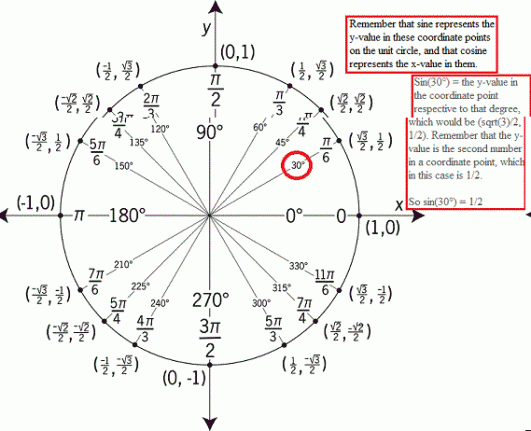sin(15 degree+x)+sin(45 degree-x)=1 What does x equal?

Sin(15°+x) + Sin(45°-x) = 1

To start solving this problem, you must use the sum-to-product formula* for the addition of two sine terms, where sin(a) + sin(b) = 2sin[(a+b)/2]cos[(a-b)/2]. In this case, the value for "a" is 15°+x and the value for "b" is 45°-x. So let's plug these values in, shall we?

2sin[(15°+x)+(45°-x) /2]cos[(15°+x)-(45°-x) /2] = 1

Simplify the terms inside the parenthesis for both the sine and cosine values. In (15°+x)+(45°-x), since we're adding them, we can directly add 15 with 45 to give 60 degrees. And when we add x with negative x, that just cancels out to 0, so 60 + 0 is still 60. And we're also dividing that entire sum by 2, so 60 divided by 2 is equal to 30 degrees.

2sin(30°)cos[(15°+x)-(45°-x) /2] = 1

For (15°+x)-(45°-x), notice there's some subtraction going on. We're going to distribute that to 45 degrees and -x, like so:

2sin(30°)cos(15°+x-45°+x /2) = 1

Notice how the 45° was originally positive, but turned negative since we distributed negative sign to it as well as to the -x. When you add a negative to something that's already negative, it turns positive, so -x turns into positive x. Now let's combine them.

15° minus 45° turns into -30°, and x+x turns into 2x, so we have 2x - 30°. But we're also dividing that entire difference by 2, so (2x-30°)/2 = x-15°. Now we have:

2sin(30°)cos(x-15°) = 1

Oh look, we have sin(30°) in the equation. Cool, let's check out what the value of it is on the unit circle.Now that we know that sin(30) is 1/2, let's look back at our equation.

2sin(30°)cos(x-15°) = 1

If we plug in 1/2 for sin(30), we also have to multiply it by 2, so 1/2 * 2 is equal to 1. And 1 times cos(x-15) is just cos(x-15), so we will have:

cos(x-15) = 1

Now think about this, what degree must cosine have to equal exactly to 1? Remember that on a unit circle, the horizontal axis represents values for x. The circle stretches to x values -1 and 1 from left to right, as well as y-values -1 to 1 from bottom to top. Since cosine focuses on x-values, pay attention to the horizontal axis. Where would cosine of anything equal to 1?

Answer: Cosine of something equals to 1 when that something is at 0 degrees, or 360n degrees. You see, when we have cos(0) the coordinate point that's respective to 0 degrees is (1,0), and since we know that cosine focuses on x-values, our answer to cos(0) will be 1. So cos(0) = 1.

Ofcourse, you could always just add 360 to it, because it will give you the same answer. The unit circle expands to a full 360 degrees, so going around the whole circle once will lead you back to where you started. So other valid choices could be cos(360), cos(720), cos(1080), etc. (That's where 360n comes in. The "n" represents how many times you add 360, so it has to be some whole number).

Alright, so let's look back at our equation. We have:

cos(x-15) = 1

So what value does x have to be in order to get cos(0), since we now know that cos(0) = 1? Well it's easy, just set x - 15 equal to 0 and solve for x.

x - 15 = 0

x = 15 (added 15 to both sides)

So x equals to 15 degrees. But remember, you can also add in any amount of 360 to it because it will give you the same answer, since adding 360 degrees will mean you'll go around the entire unit circle once and end up back where you started. So the real answer here is

x = 15° + 360°n

1 Person thanked the writer.
View all 5 Comments
John McCann commented
Don't worry about it too much as it is not like I am overwhelmed here. The science and math sections are frozen for days now. I am not sure why but I suspect it might have something to do with people coming from other sites that folded up. They seem to be against answering HW questions. When ask.com folded we had a rash of those negative answers.

Not one of these people who pooh pooh HW questions could even approach a problem like this in their wildest dreams!
David Shabazi commented
Yeah. Some people who are against answering questions like these provide "negative" answers because they want the person who asked it to figure it out on their own, and hopefully learn that way.

But the thing is, the person who asked the question could have tried everything possible to figure it out on their own, but they just couldn't do it. It's not clicking for them. So naturally they seek assistance from others who (hopefully) can help them. Most of the time, it's a matter of miscommunication (from the teacher) or misunderstanding (from either the teacher or the textbook), and it leaves the student confused from the start, so in turn they'll have no idea how to go about answering a question like this. But I'm glad I get the opportunity to actually show them how to do it from start to finish. If this was a homework question, then technically yes I did a very tiny portion of their homework for them, but I've also explained the steps I've taken in detail so that they can replicate it for future similar questions.
John McCann commented
Exactly my thoughts on the subject. Math especially can be taught analogously, one problem worked for a person leads to a whole class of problems becoming tractable to that person.
Some sites have guidelines against HW questions and I have a few of those that I hardly go to anymore. Some site develop a tradition of anti-HW and those I rarely go to either.